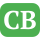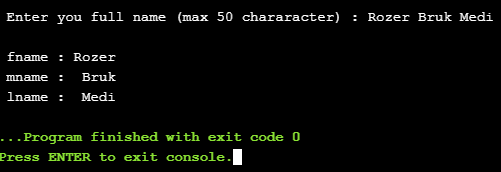CppBuzz.com

# C program to split full name into First, Middle and Last Name

This C program takes fullname from user and split it into First, Middle & Last Name

``````#include <stdio.h>

int main()
{
char name = "\0";
char fname = "\0";
char mname= "\0";
char lname= "\0";

char * ptr = NULL;
int count =0;
int i,j,k,l;
j=k=l=0;

printf("\n Enter you full name (max 50 chararacter) : ");
gets(name);

for(i=0; i<strlen(name); i++){

if(name[i] == ' ')
count++;

if(count ==0)
fname[j++] = name[i];
if(count ==1)
mname[k++] = name[i];
if(count ==2)
lname[l++] = name[i];

}

printf("\n fname : %s", fname);

if(strlen(lname)==0){
printf("\n lname : %s", mname);
}
else{
printf("\n mname : %s", mname);
printf("\n lname : %s", lname);
}

return 0;
}
``````